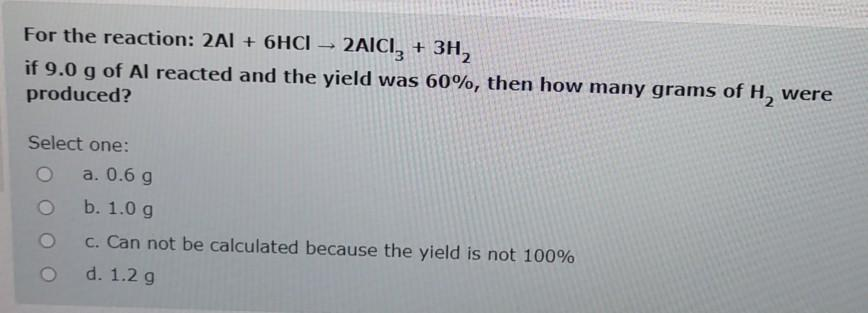### Create an Account

Home / Questions / For the reaction: 2A1 + 6HCI – 2AICI; + 3H, if 9.0 g of Al reacted and the yield was 60%, ...

# For the reaction: 2A1 + 6HCI – 2AICI; + 3H, if 9.0 g of Al reacted and the yield was 60%, then how many grams of H, were produced? Select one: a. 0.6 g b. 1.0 g c. Can not be calculated because the

For the reaction: 2A1 + 6HCI – 2AICI; + 3H, if 9.0 g of Al reacted and the yield was 60%, then how many grams of H, were produced? Select one: a. 0.6 g b. 1.0 g c. Can not be calculated because the yield is not 100% d. 1.2 gMay 20 2021 View more View LessSubscribe To Get Solution